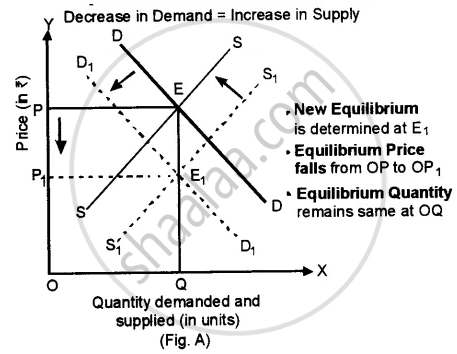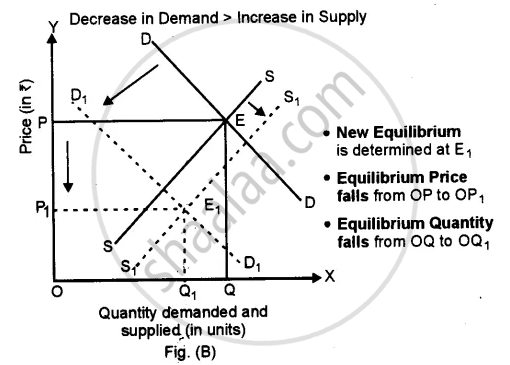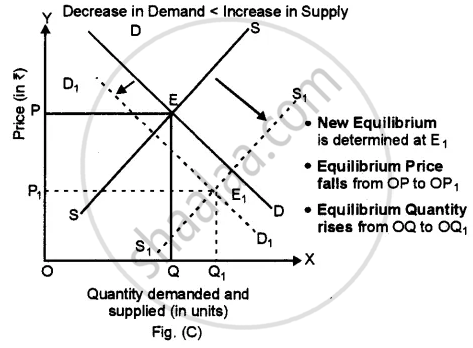# Answer the Following Question. Show with the Help of Diagrams, the Effect on Equilibrium Price and Quantity When (I) There is a Fall in the Price of Substitute Goods. (Ii) There is a Rise in the Price - Economics

Show with the help of diagrams, the effect on equilibrium price and quantity when:
There is a fall in the price of substitute goods.

#### Solution

1. A decrease in the price of a substitute good will disturb the equilibrium point and quantity of the commodity.
2. Due to a decrease in the price of a substitute good (say coffee) demand for tea will fall because tea has become relatively costly.
3. A decrease in the price of a substitute good will have a direct impact on the supply (supply will increase) of the good because produces of tea will like to clear their stock as fast as possible.
4. The new equilibrium can be studied in three different cases.

Case 1: Decrease in Demand = Increase in Supply
When the decrease in demand is proportionately equal to the increase in supply, then the leftward shift in demand curve from DD to D1D1 is proportionately equal to a rightward shift in the supply curve from SS to S1S1.
(Fig. A). The new equilibrium is determined at E1. As the decrease in demand is proportionately equal to the increase in supply, equilibrium quantity remains the same at OQ, but equilibrium price falls from OP to OP1.Case 2: Decrease in Demand > Increase in Supply
When a decrease in demand is proportionately more than an increase in supply, then the leftward shift in demand curve from DD to D1D1 is proportionately more than the rightward shift in the supply curve from SS to S1S1 (Fig. B). The new equilibrium is determined at E1. As the decrease in demand is proportionately more than the increase in supply, equilibrium quantity falls from OQ to OQ1 and equilibrium price falls from OP to OP1.Case 3: Decrease in Demand < Increase in Supply
(i) When the decrease in demand is proportionately less than an increase in supply, then the leftward shift in demand curve from DD to D1D1 is proportionately less than a rightward shift in supply curve from SS to S1S1 Fig. C). The new equilibrium is determined at E1. As the decrease in demand is proportionately less than the increase in supply, equilibrium quantity rises from OQ to OQ1 whereas, equilibrium price falls from OP to OP1.Concept: Market Equilibrium
Is there an error in this question or solution?
2014-2015 (March) Set 1

Share